# First-in, first-out (FIFO) method in periodic inventory system

Under first-in, first-out (FIFO) method, the costs are chronologically charged to cost of goods sold (COGS) i.e., the first costs incurred are first costs charged to cost of goods sold (COGS). This article explains the use of first-in, first-out (FIFO) method in a periodic inventory system. If you want to read about its use in a perpetual inventory system, read “first-in, first-out (FIFO) method in perpetual inventory system” article.

In a periodic inventory system when a sale is made, the entry to record the cost of goods sold is not made. At the end of accounting period, the quantity of inventory on hand (ending inventory) is found by a physical count and if the FIFO method is used to compute the cost of ending inventory, the cost of most recent purchases are used. Once the cost of ending inventory has been computed, the cost of goods sold can be computed easily using the following simple formula:

Cost of goods sold (COGS) = Beginning inventory + Purchases – Ending inventory

The following example illustrates the use of FIFO method in a periodic inventory system:

## Example:

The Sunshine company uses periodic inventory system. The company makes a physical count at the end of each accounting period to find the number of units in ending inventory. The company then applies first-in, first-out (FIFO) method to compute the cost of ending inventory.

The information about the inventory balance at the beginning and purchases made during the year 2016 are given below:

• Mar. 01: Beginning balance; 400 units @ \$18 per unit.
• Mar. 12: Purchases; 600 units @ \$20 per unit.
• Oct. 17: Purchases; 800 units @ \$22 per unit.
• Dec. 15: Purchases; 200 units @ \$24 per unit.

On 31st December 2016, 600 units are on hand according to physical count.

Required: Compute the following using first-in, first-out (FIFO) method:

1. Cost of ending inventory at 31 December 2016.
2. Cost of goods sold during the year 2016.

### Solution:

#### (1). Cost of ending inventory – FIFO method:

If FIFO method is used, the units remaining in the inventory represent the most recent costs incurred to purchase the inventory. The cost of 600 units on 31 December would, therefore, be computed as follows: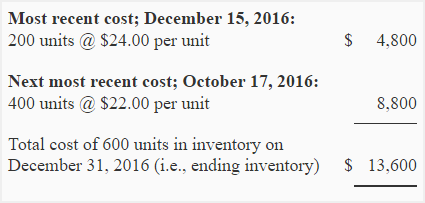#### (2). Cost of goods sold – FIFO method

Cost of goods sold can be computed by using either periodic inventory formula method or earliest cost method.

a. Formula method: Under formula method, the cost of goods sold would be computed as follows:

Cost of goods sold = Cost of units in beginning inventory + Cost of units purchased during the period – Cost of units in ending inventory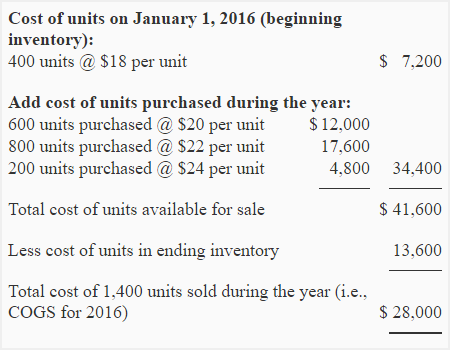b. Earliest cost method: Under earliest cost method, we would find the total number of units sold during the period and then we would calculate the cost of these units using earliest costs.

Number of units sold = Beginning inventory + Purchases – Ending inventory

= 400 units + 1,600* units – 600 units

= 1,400 units

*600 + 800 + 200

The 1,400 units sold during the year would be costed using earliest costs as follows: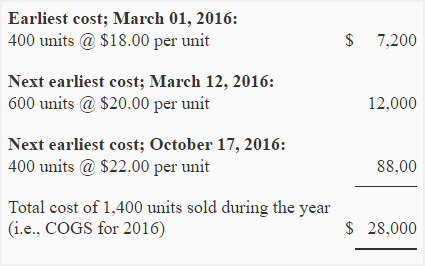The periodic inventory and FIFO concepts can also be applied for recording and valuing direct materials in manufacturing companies. To understand their use in a manufacturing company, consider the following example:

## Example:

The Galaxy manufacturing company has provided the following information about beginning balance and purchases of direct material for the year 2016:

• 01 Jan: Beginning balance; 2,800 units @ \$9.20 per units.
• 15 Apr: Purchases; 2,000 units @ \$9.50 per units.
• 20 Aug: Purchases; 2,500 units @ \$9.80 per units.
• 15 Nov: Purchases; 1,000 units @ \$10.20 per units.

At the end of the year 2016, the company makes a physical measure of material and finds that 1,700 units of material is on hand.

Required:

1. Compute the cost of materials on hand at the end of the year.
2. Also compute the cost of materials issued to production during the year.

#### (1). Cost of materials on hand at the end of the year  – FIFO method: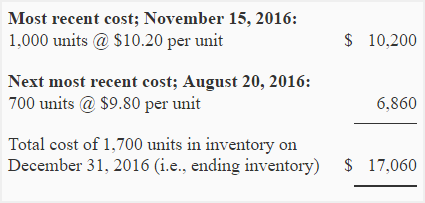#### (2). Cost of materials issued for production during the year – FIFO method:

a. Formula method: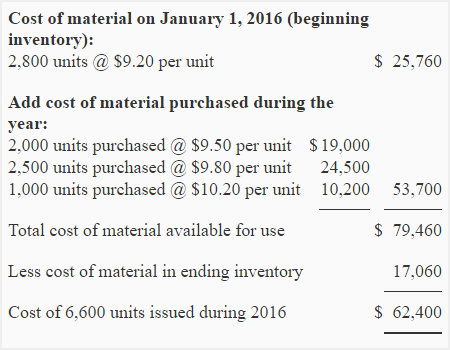b. Earliest cost method:

Number of units issued = Units in beginning inventory + Units purchased during the period – Units in ending inventory

= 2,800 units + 5,500* units – 1,700 units

= 6,600 units

*2,000 + 2,500 + 1,000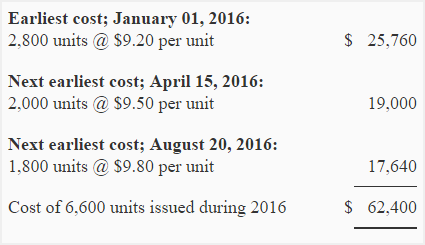Show your love for us by sharing our contents.

### 28 Comments on First-in, first-out (FIFO) method in periodic inventory system

1.nick

1.agreed

2.jane

how are arriving at 400 next and 700 kindly explain

2.p sundar

It definitely is useful

3.4.Vetyeka

In example no.2 The galaxy manufacturing…
where did you get the next most recent cost: 700 pounds and it’s amount of \$9.80?

thank you.

5.Accounting For Management

The Galaxy manufacturing company has 1,700 pounds of raw materials on hand at the end of the year. FIFO periodic inventory system assumes that 1,000 pounds are from purchases made on 15 November and remaining 700 (1,700 – 1,000) are from purchases made on 20 August. So the ending inventory can be computed as follows:

(1,000 pounds × \$10.20) + (700 pounds × \$9.80)
= \$17,060

The company has issued a total of 6,600 pounds of materials to production. The cost of total materials issued to production can be computed as follows:

(2,800 pounds × \$9.20) + (2,000 pound × \$9.50) + (1,800 pound × \$9.80)
= \$62,400
or
Beginning inventory + Purchases – Ending inventory
\$25,760 + \$53,700 – \$17,060
= \$62,400

Hope this helps.

6.moses

we are assume that 1700 =100 and 700. my question is that how did we get the 10.20 for \$100 and 9.80 for \$700. thank you.

7.Accounting For Management

Because we are using FIFO method.
Under first-in first-out (FIFO) method of inventory valuation we assume that the ending inventory of 1,700 units consist of 1,000 units purchased on 15 November and 700 units (out of 2,500 units) purchased on 20 August.

8.Joyce Monjimbo

Hello mister,

I would like to thank you for your explanation. For a couple of days I have been struggling to understand how to calculate ending inventory and you have elucidated the confusion I had. I asked my professor to help me but I guess since I didn’t phrase my question clearly she misunderstood what I expected as a reply. Once again thanks. Now I know how to figure out the ending inventory using the periodic method.

Joyce.

9.mpho

10.mpho

it is very helpful and useful

11.hl

What if when counting inventory at the end of the year, the company finds that there are less inventory on hand? What would happen then?

12.serbey

when computing for ending inventory, what if the ending purchases is greater than the purchases before, is there any negative purchases??

13.Accounting For Management

It is ending inventory, not ending purchases. The inventory that you purchased but remained unsold.

14.nemai raralevu

hi sir,

i still dont understand how to calculate the ending inventory

15.Helen

Hi, I’m pretty sure FIFO is the first-in first-out cost assumption, which means that the oldest inventory should go out first instead of the most recent which is LIFO.

16.Fredrick

very useful,though am Kenyan but I find it so useful to me

17.Fredrick

how can you calculate a sum where you are required to use FIFO method to determine the value of inventory but in the sales column you’ve been given the amount your are to sell the units of the first consignment manufactured? I find it not applicable coz if am not wrong one of the rules of FIFO is consignment are to be dispersed/sold at the same price they were brought in…if need be I can give the quizz in this forum

18.Engr Ghulam Mustafa

very nice for simple understanding

19.Mubala remigius

how do i get the rate at wich materails were issued or sold?

20.Principles of Financial Accounting

Pretty sure this is backwards. FIFO(First in first out) is when all goods sold are priced under the same as the first goods purchased. The inventory sold also begins with the first inventory bought and moves progressively more towards recent purchases as stock of those purchases is used up.

21.janardan panday

It is simple to understand but in the situation of the receipt of free inventory (bonus) in lieu of the purchased stock in earlier date,If purchased inventory is sold and free bonus only remains in store the cost of the bonus will be 0 despite of the fact that free bonus was on the basis of purchased inventory and hidden cost is also there.Hence the FIFO method of accounting seem to be unrealistic may be convenient.

22.can someone help me with this one
If a company has the following inventory records:
January 1 On hand, 100 units @ \$100 each from BatchA
19 Purchased 100 units @ \$ 120 each from Batch C

And counted it’s inventory at the end of January finding 70 there

What would it record as closing inventory of it was using the three common methods?

23.tman

how to u calculate cost price per unit of stock on hand?(FIFO method)

24.MAHESH VERMA

25.26.